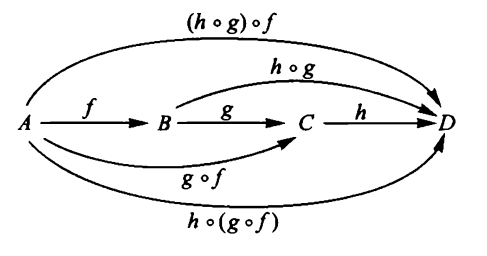# nLab associativity

Contents

category theory

## Applications

#### Higher category theory

higher category theory

# Contents

## Definition

In a category associativity is the condition that the two ways to use binary composition of morphisms to compose a sequence of three morphisms are equal

$h \circ (g \circ f) = (h \circ g) \circ f \phantom{AAAA}$$\array{ c_2 &\overset{ \phantom{AA}g\phantom{AA} }{\longrightarrow}& c_3 \\ {}^{\mathllap{f}}\Big\uparrow & \searrow^{\mathrlap{h \circ g}} & \Big\downarrow{}^{\mathrlap{h}} \\ c_1 &\underset{ (h \circ g) \circ f }{\longrightarrow}& c_4 } \;\;\;\;=\;\;\;\; \array{ c_2 &\overset{ \phantom{AA}g\phantom{AA} }{\longrightarrow}& c_3 \\ {}^{\mathllap{f}}\Big\uparrow & {}^{\mathllap{ g \circ f }}\nearrow & \Big\downarrow{}^{\mathrlap{h}} \\ c_1 &\underset{ h \circ (g \circ f) }{\longrightarrow}& c_4 }$

If the category has a single object it is the delooping $\mathcal{B} A$ a monoid $A$, and then this condition is the associativity condition on the binary operation of monoids such as groups, rings, algebras, etc.

More generally, in higher category theory, associativity of composition of morphisms in an n-category means that the different ways to use binary composition for composing collections of k-morphisms form a contractible infinity-groupoid. This is a coherence law.

For instance the associativity law in an A-infinity algebra is the special case of associativity in a 1-object A-infinity-category.

## Examples

The coherence law of associativity is stated in